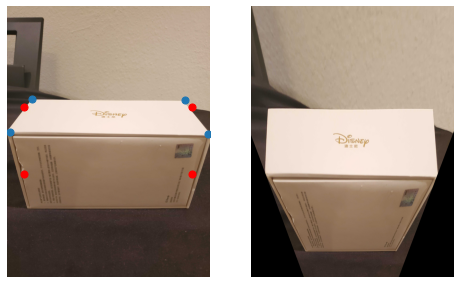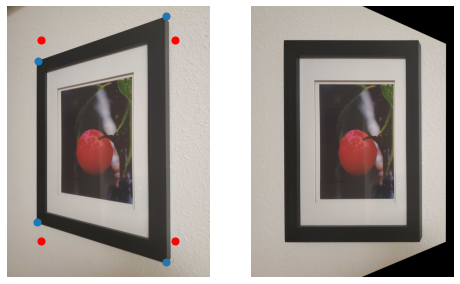In :
from skimage import color, transform, filters
import skimage.io as skio
import numpy as np
import os
import numpy as np
import matplotlib
import matplotlib.pyplot as plt
import seaborn as sns
import pickle
import cv2

# Ignore warnings
import warnings
warnings.filterwarnings("ignore")


# Project 5A: Image Warping and Mosaicing

David Yi

## Shoot and Digitize Pictures¶

My goal for the first part of the project was to create image panoramas through manual homographic projections. I took the pictures below near my house with locked aperture and exposure camera settings, but the pictures were taken on my smartphone so the point of view may not necessarily be constant. Regardless, the perspective transforms turned out fairly well, as you will see in later parts.In :
matplotlib.use('module://ipykernel.pylab.backend_inline')
# matplotlib.use('TkAgg')

# plt.imshow(img)
# pts = plt.ginput(n=13, timeout=0)

# with open('garage_r.p', 'wb') as fp:
#     pickle.dump(pts, fp)


## Recovering Homographies¶

The homography transform between a set of corresponding points between two images is defined by:

$p' = Hp$, where $H$ is a $3x3$ matrix with 8 unknowns. We only need four equations (pairs of correspondence points) to recover the 8 unknowns, but the recovery may be unstable and prone to noise. Alternatively, we can solve an overdetermined system by providing more than 4 correspondences and rearranging $H$ into a $9x1$ vector. Our new system is $PH = \vec{0}$, where $P$ is the $Nx9$ matrix composed by stacking the following submatrix $p_i$ for each correspondence pair. source: http://6.869.csail.mit.edu/fa12/lectures/lecture13ransac/lecture13ransac.pdf

\begin{equation*} p_i = \begin{bmatrix} -x_i & -y_i & -1 & 0 & 0 & 0 & x_ix_i' & y_ix_i' & x_i' \\ 0 & 0 & 0 & -x_i & -y_i & -1 & x_iy_i' & y_iy_i' & y_i' \end{bmatrix} \end{equation*}

and

\begin{equation*} H = \begin{bmatrix} h_1 \\ h_2 \\ h_3 \\ h_4 \\ h_5 \\ h_6 \\ h_7 \\ h_8 \\ h_9 \end{bmatrix} \end{equation*}

where the least squares solution to $H$ is the right singular vector corresponding to the smallest eigenvalue of the Singular Value Decomposition of $P$

In :
def computeH(im1_pts, im2_pts):
P = np.empty((0, 9))
for i in range(len(im1_pts)):
im1 = im1_pts[i]
im2 = im2_pts[i]
p_1 = np.array([-im1, -im1, -1, 0, 0, 0, im1*im2, im1*im2, im2])
p_2 = np.array([0, 0, 0, -im1, -im1, -1, im1*im2, im1*im2, im2])
P = np.vstack((P, p_1.reshape(1, 9)))
P = np.vstack((P, p_2.reshape(1, 9)))
return np.linalg.svd(P)[-1].reshape(3, 3)

In :
def warpImage(im, H):
H_inv = np.linalg.inv(H)
H_inv = H_inv / np.sqrt(np.sum(H_inv**2))
h, w = im.shape[:2]
indy, indx = np.indices((h, w), dtype=np.float32)
lin_homg_ind = np.array([indx.ravel(), indy.ravel(), np.ones_like(indx).ravel()])

# warp the coordinates of src to those of true_dst
map_ind = H_inv @ lin_homg_ind
map_x, map_y = map_ind[:-1]/map_ind[-1]  # ens|ure homogeneity
map_x = map_x.reshape(h, w).astype(np.float32)
map_y = map_y.reshape(h, w).astype(np.float32)

# remap!
dst = cv2.remap(im, map_x, map_y, cv2.INTER_LINEAR)
return dst


### Image Rectification (Warping)¶

Although I warp all of my left images to align with the right image, I actually use the inverse Homography matrix $H^{-1}$ to perform an inverse warp and fill in pixels using linear interpolation. Before rectifying the images shown above, I test that my homography matrix $H$ is correct by "rectifying" images to be front-parallel by guessing the correspondence points. The "guesses" are shown as blue dots in the original image.

In :
box = skio.imread('box.jpg')
matplotlib.use('module://ipykernel.pylab.backend_inline')
matplotlib.use('TkAgg')

# plt.imshow(box, cmap='gray')

# pts = plt.ginput(n=4, timeout=0)

# with open('box_p.p', 'wb') as fp:
#     pickle.dump(pts, fp)

In :
matplotlib.use('module://ipykernel.pylab.backend_inline')
with open('box_p.p', 'rb') as fp:

new_pts = np.array([(250, 1500), (2750, 1500), (250, 2500), (2750, 2500)])

f,axs = plt.subplots(1, 2, figsize=(8, 8))

plt.subplot(1, 2, 1)
plt.imshow(box, cmap='gray')
plt.scatter(box_p[:, 0], box_p[:, 1], s=50)
plt.scatter(new_pts[:, 0], new_pts[:, 1], s=50, color='r')
plt.axis('off')

plt.subplot(1, 2, 2)
H = computeH(box_p, new_pts)
plt.imshow(warpImage(box, H), cmap='gray')
plt.axis('off');In :
frame = skio.imread('frame.jpg')

matplotlib.use('module://ipykernel.pylab.backend_inline')
matplotlib.use('TkAgg')

# plt.imshow(frame, cmap='gray')

# pts = plt.ginput(n=4, timeout=0)

# with open('frame_p.p', 'wb') as fp:
#     pickle.dump(pts, fp)

In :
matplotlib.use('module://ipykernel.pylab.backend_inline')
with open('frame_p.p', 'rb') as fp:

new_pts = np.array([(500, 500), (2500, 500), (500, 3500), (2500, 3500)])

f,axs = plt.subplots(1, 2, figsize=(8, 8))

plt.subplot(1, 2, 1)
plt.axis('off')
plt.imshow(frame, cmap='gray')
plt.scatter(frame_p[:, 0], frame_p[:, 1], s=50)
plt.scatter(new_pts[:, 0], new_pts[:, 1], s=50, color='r')b

plt.subplot(1, 2, 2)
plt.axis('off')
H = computeH(frame_p, new_pts)
plt.imshow(warpImage(frame, H), cmap='gray');These were fairly simple examples, let us now put our algorithm to the test by creating mosaics from our original images!

## Blending the Images into a Mosaic¶

My process of creating an image is as follows:

1. Manually assign correspondence points between the left and right image
2. Create "bounding box" for left image by warping the corner points with the homography matrix $H$.
3. Recompute the homography matrix $H$ and the correspondence points to account for the displacement from padding
4. Warp the left image to align with the right image
5. Combine the two images by taking the maximum pixel between overlapping points and crop!

Below, I show the rectified image on the left and the final panoramic image on the right.

The algorithm works fairly well, although we can definitely see small issues with blurriness and brightness in some of the overlapping sections.

In :
with open('window_l.p', 'rb') as fp:
with open('window_r.p', 'rb') as fp:

In :
# matplotlib.use('module://ipykernel.pylab.backend_inline')

# plt.imshow(window_l)
# plt.scatter(window_lp[:, 0], window_lp[:, 1], c='r')

In :
# plt.imshow(window_r)
# plt.scatter(window_rp[:, 0], window_rp[:, 1], c='r')

In :
H = computeH(window_lp, window_rp)

coords = np.zeros((0, 2))
points = np.array([[0, 0, 1], [4032, 0, 1], [0, 3024, 1], [4032, 3024, 1]])

for p in points:
new_p = H @ np.array(p)
new_p = new_p[:2] / new_p
coords = np.vstack((coords, new_p))

new_x = int(np.max(coords[:, 0]) - min(np.min(coords[:, 0]), 0)) + 2000
new_y = int(np.max(coords[:, 1]) - min(np.min(coords[:, 1]), 0))

In :
x_diff = int((new_x - window_l.shape)/2)
y_diff = int((new_y - window_l.shape)/2)

f, axs = plt.subplots(1, 2, figsize=(20, 15))

plt.subplot(1, 2, 1)
plt.axis('off')

window_lp[:, 0] += x_diff
window_lp[:, 1] += y_diff
window_rp[:, 0] += x_diff
window_rp[:, 1] += y_diff
new_H = computeH(window_lp, window_rp)